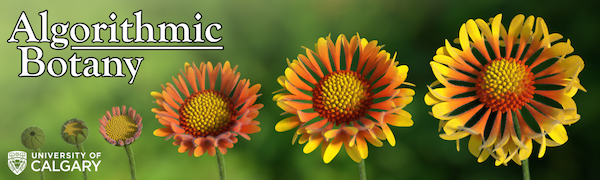# CPSC 453

## Introduction to Computer Graphics

### Fall 2019

• Lectures
• Lecture 1: Introduction (PPT) (PDF)
• Lecture 2: Mathematical background (PPT) (PDF)
• Lecture 3: Graphics output devices (PPT) (PDF)
• Lecture 4: Software/hardware interface
• The rendering pipeline (PPT) (PDF)
• A programming example (PPT) (PDF)
• Lecture 5: Transformations in 2D (no additional materials)
• Lecture 6: Transformations in 3D; examples of transformations in 2D and 3D; vector libraries
• Derivation of Rodrigues' formula (PDF)
• Programming examples:
• On (my) Mac, these files compile using commands such as:
• clang -framework OpenGL -framework GLUT rodrigues.cpp -o rodrigue
• Lecture 7: Viewing transformation
• Screen, camera and orthographic transformation (PPT) (PDF)
• Lecture 8: Perspective (PDF) (PPT)
• Lecture 9: Rasterization
• Line and triangle rasterization, clipping (PDF)
• Lecture 10: Polygon meshes (Textbook chapters 12.1.1-12.1.3, no additional materials)
• Lecture 12-13: Texture mapping (PPT) (PDF)
• Lecture 14: Material review
• Lecture 15: Midterm
• Lecture 16: Midterm results review
• Lecture 17-19: Ray tracing (Textbook Chapters 4 and 13; no additional materials)
• Lecture 20-21: Subdivision curves (PDF)
• Lecture 22-23: Parametric curves and surfaces (PDF)
• Lecture 24: Subdivision surfaces + a note on other modeling methods
• Lecture 25: Final review (DOC) (PDF)
• Assignments
1. Introduction to programming with OpenGL: generating and viewing the Menger sponge (DOC) (PDF)
2. A 3D Object Viewer (DOC) (PDF)
• Updated due data: October 29, 2019
• Sample models. See assignment description for sample images.
• teapot (ZIP)
• spot (ZIP)
• Nefertiti (Low polygon) (ZIP)
• Nefertiti (High polygon) (UPDATED Oct. 21) (ZIP)
3. Ray-tracing (DOC) (PDF)
4. Modeling Updated due data: December 8, 2019 (DOC) (PDF)
• Tutorials
• Tutorial 1: Math review (PDF)
• Tutorial 2: C++ review and sample code (PDF, ZIP )
• Tutorial 3: OpenGL 2.0 and First triangle code (PDF, TARGZ, Solution TARGZ )
• Tutorial 4: OpenGL 3.3+ and First triangle (again with OpenGL 3.3) (PDF, TARGZ )
• Tutorial 5: Sierpinski Triangle (TARGZ )
• Tutorial 6: Matrices and transformations (UPDATED FOR MAC OS) (ZIP )
• Tutorial 7: Demos
• Tutorial 8: Self test